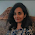VHDL coding tips and tricks: Triangular Wave generator in VHDL

## Friday, April 24, 2015

### Triangular Wave generator in VHDL

Triangle wave look like this:

A simple triangle wave generator was designed in VHDL. You cannot change the frequency of the wave, without changing the input frequency. The precision is fixed at 8 bits 2's complement format. But if want more precision you can increase the size of the register.

Triangle wave vhdl code:

library IEEE;
use IEEE.STD_LOGIC_1164.ALL;
use IEEE.STD_LOGIC_ARITH.ALL;
use IEEE.STD_LOGIC_UNSIGNED.ALL;

entity triangular is
port (clk : in std_logic;
wave_out : out std_logic_vector(7 downto 0);
reset :in std_logic
);
end triangular;

architecture Behavioral of triangular is

signal count,count2 : integer := 0;
signal direction : std_logic := '0';

begin

process(clk,reset)
begin
if(reset = '1') then
count <= 0;
count2 <= 129;
elsif(rising_edge(clk)) then
--"direction" signal determines the direction of counting - up or down
if(count = 253) then
count <= 0;
if(direction = '0') then
direction <= '1';
count2 <= 126;
else
direction <= '0';
count2 <= 129;
end if;
else
count <= count + 1;
end if;
if(direction = '0') then
if(count2 = 255) then
count2 <= 0;
else
count2 <= count2 + 1; --up counts from 129 to 255 and then 0 to 127
end if;
else
if(count2 = 255) then
count2 <= 0;
else
count2 <= count2 - 1; --down counts from 126 to 0 and then 255 to 128
end if;
end if;

end if;
end process;

wave_out <= conv_std_logic_vector(count2,8);

end Behavioral;

Testbench code:

library IEEE;
use IEEE.STD_LOGIC_1164.ALL;
use IEEE.STD_LOGIC_ARITH.ALL;
use IEEE.STD_LOGIC_UNSIGNED.ALL;

entity tb_triangular is
end tb_triangular;

architecture Behavioral of tb_triangular is

component triangular is
port (clk : in std_logic;
wave_out : out std_logic_vector(7 downto 0);
reset :in std_logic
);
end component;

signal clk,reset : std_logic := '0';
signal wave_out : std_logic_vector(7 downto 0);

begin

uut : triangular port map(Clk,wave_out,reset);

Clk <= not Clk after 5 ns;

process
begin
reset <= '1';
wait for 100 ns;
reset <= '0';
wait;
end process;

end Behavioral;

The code was simulated using modelsim. You should get the following waveform when you simulate the codes correctly:

To see the output wave in analog shape,as above, you need to change the signal format into analog(automatic).

#### 3 comments:

1.ProjectMgmt:806 - "F:/aprojct/ritu/ritttuu/ttr_1.vhd" Line 60. Syntax error near "Testbench".

how to solw it?

2.Why are you intiallize the count2 with 126 and 129 only

3.I have an error :
Signal count cannot be synthesized, bad synchronous description. The description style you are using to describe a synchronous element (register, memory, etc.) is not supported in the current software release.
-->
how to do please !